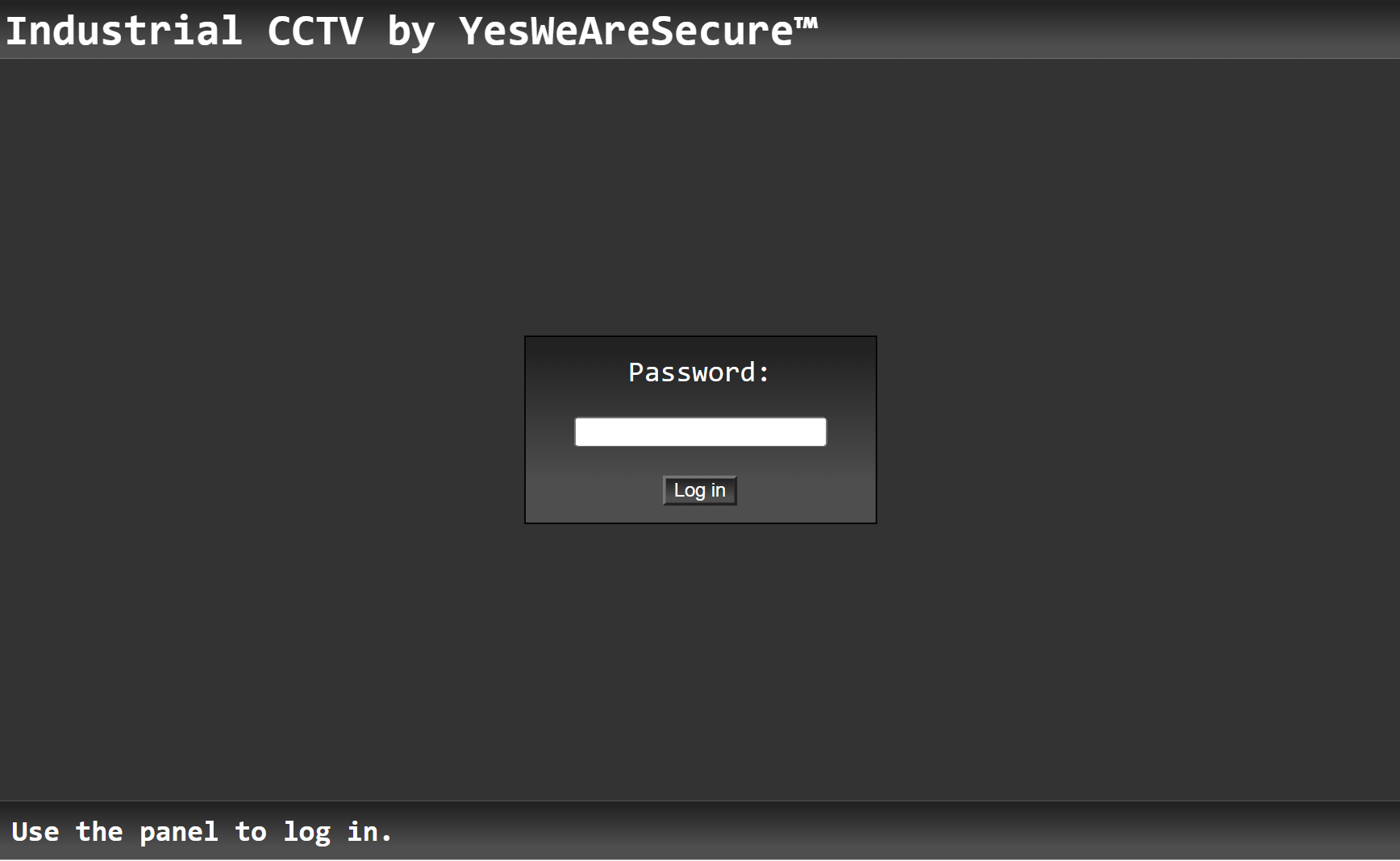WebReversing

1 year ago - 320 views

# 1 - CCTV

The first challenge gives us a link (https://cctv-web.2021.ctfcompetition.com/) to a login page.Putting a random password in here gives us an alert that the password is wrong.

## Analysing

Since this is tagged as a reversing challenge, it is always a good idea to look at the underlying code. By pressing Ctrl+U you can view the source code of the page, and scroll down a bit.
Here we see the following JavsScript code:

JavaScript

``````const checkPassword = () => {
const p = Array.from(v).map(a => 0xCafe + a.charCodeAt(0));

if(p === 52037 &&
p === 52081 &&
p === 52063 &&
p === 52077 &&
p === 52077 &&
p === 52080 &&
p === 52046 &&
p === 52066 &&
p === 52085 &&
p === 52081 &&
p === 52077 &&
p === 52066) {
window.location.replace(v + ".html");
} else {
}
}``````

Looking at this code it seems the password is being checked with the condition in the `if` statement. It alerts us when the password is wrong and changes the URL to the inputted password if we get it right.
Our inputted password doesn't go raw into the condition, it first gets mapped by the
`const p = Array.from(v).map(a => 0xCafe + a.charCodeAt(0));`
function. This code just adds the hexidecimal value `0xcafe` to every individual character's ascii value. Then it places them all in the array `p`.

## Solution

Now we need to create a password the passes all these checks.
We can just do the reverse of these steps, to end up with the password. By subtracting `0xcafe` instead of adding it we can get the original value. Then we need to just place the letters in the correct order to get the password.
We can easily get all the `p` values from the condition in an array like this:

JavaScript

``````p = []
p = 52037
p = 52081
p = 52063
p = 52077
p = 52077
p = 52080
p = 52046
p = 52066
p = 52085
p = 52081
p = 52077
p = 52066``````

Then we need to map these values in reverse, back to the original value. By just subtracting the `0xcafe` we get that value.

JavaScript

``````o = p.map(a => String.fromCharCode(a - 0xCafe));  // Map values back in reverse
console.log(o.join(''))  // Log output as string instead of array``````

This logs `GoodPassword` to the console, and trying to input this as a password to the login page redirects us to a page with four CCTV cameras, with the flag on the bottom!
`CTF{IJustHopeThisIsNotOnShodan}`